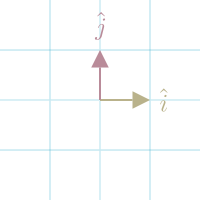## Basis VectorsThe set of Vectors that defines space is called the basis.

We refer to these vectors as basis vectors.

In 2d space, basis vectors are commonly defined as $\hat{i} = \begin{bmatrix}1 \\ 0\end{bmatrix}$ and $\hat{j} = \begin{bmatrix}0 \\ 1\end{bmatrix}$.

These particular vectors are called the standard basis vectors. We can think of them as 1 in the direction of X and 1 in the direction of Y.

We can think of all other vectors in the space as Linear Combinations of the basis vectors.

For example, if I have vector $\begin{bmatrix}10 \\ -7\end{bmatrix}$, we can treat each component as scalar (see Vector Scaling) for the basis vectors: $10\begin{bmatrix}1 \\ 0\end{bmatrix} + (-7)\begin{bmatrix}0 \\ 1\end{bmatrix}$.

We can choose any set of vectors as the basis vectors for space, giving us entirely new coordinate systems. However, they must meet the following criteria:

• They're linear independent. That means you cannot get one Vector by just scaling the other.
• They span the space. That means, by taking a linear combination of the two scaled vectors, you can return any vector.

Basis vectors don't have to be orthogonal to each other, but transformations become more challenging with a non-orthogonal basis.

#### References

David Dye, Sam Cooper, and Freddie Page. Mathematics for Machine Learning: Linear Algebra - Home. 2018. URL: https://www.coursera.org/learn/linear-algebra-machine-learning/home/welcome.

3Blue1Brown. Linear combinations, span, and basis vectors. August 2016. URL: https://www.youtube.com/watch?v=k7RM-ot2NWY.